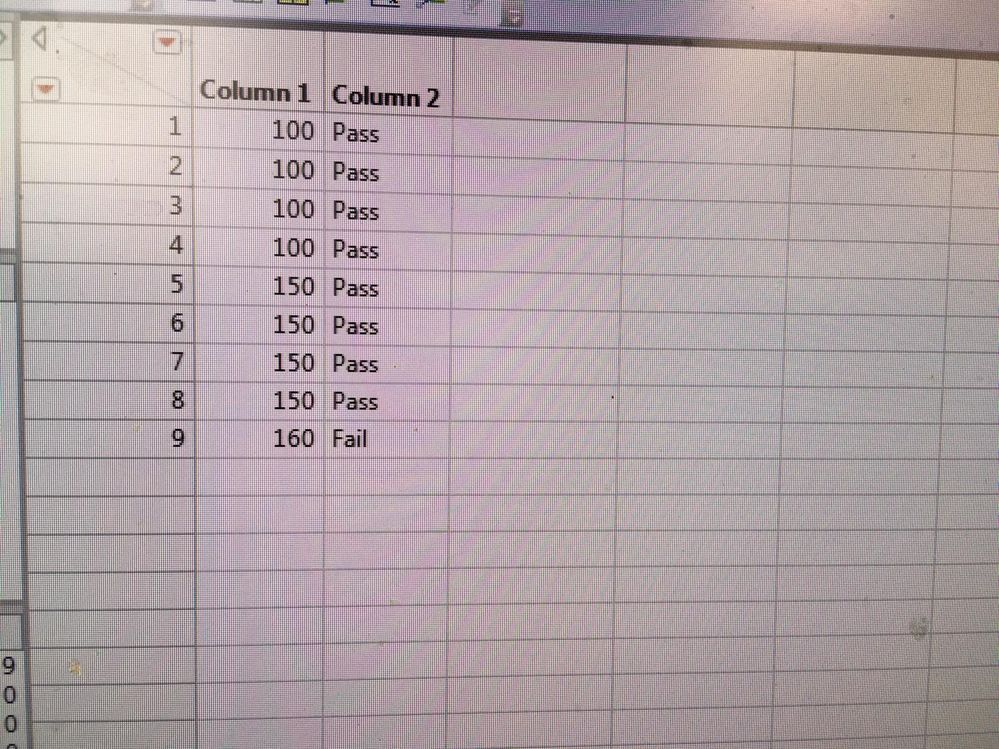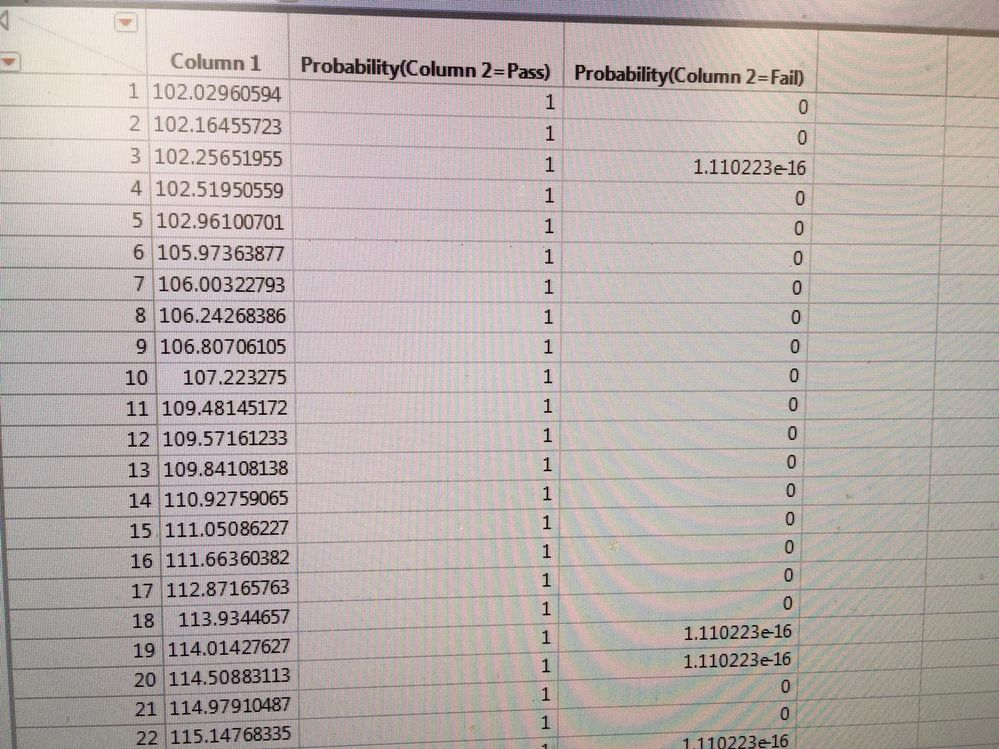Our World Statistics Day conversations have been a great reminder of how much statistics can inform our lives. Do you have an example of how statistics has made a difference in your life? Share your story with the Community!
Choose Language Hide Translation Bar
Highlighted

## Prediction Profiler - output random table

Hi All,

I have a table with 2 columns (lets say distance and pass/fail) as attached in the images of this discussion.

When I perform nominal logistic fit moudule (Y=pass/fail, X=distance), and output a random table out of the profiler. I see that for some values the failure probability is 0 and for other similar values the probability is 1E-16 (see 2'nd image attached to this discussion).A) why is that happening?

B) How can make that for all values of distance the probability will be a number other than 0 (1E-16 is fine for this example)

Thank you very much.

1 ACCEPTED SOLUTION

Accepted Solutions
Highlighted

## Re: Prediction Profiler - output random table

This may be a rather extreme case for a data set as there is a perfect fit of the data for X=100, X=150, and X=160.

You'll notice in the Parameter Estimates output that the values are 'Unstable' (it doesn't really stand out in this platform.) If you look at your Prediction Profiler, you can see that it's predicting either 0 or 1 basically for your probabilities.

When I've had logistic regression situations where at a given value of X, I have perfect fits (all pass or all fail data), I use the GLM personality in Fit Model with the Binomial Distribution and Logit link function to fit a logistic regression. This output is much more obvious about the issues with the perfect fit data, and will suggest fitting with the Firth Bias-Adjusted Estimates option checked.

2 REPLIES 2
Highlighted

## Re: Prediction Profiler - output random table

This may be a rather extreme case for a data set as there is a perfect fit of the data for X=100, X=150, and X=160.

You'll notice in the Parameter Estimates output that the values are 'Unstable' (it doesn't really stand out in this platform.) If you look at your Prediction Profiler, you can see that it's predicting either 0 or 1 basically for your probabilities.

When I've had logistic regression situations where at a given value of X, I have perfect fits (all pass or all fail data), I use the GLM personality in Fit Model with the Binomial Distribution and Logit link function to fit a logistic regression. This output is much more obvious about the issues with the perfect fit data, and will suggest fitting with the Firth Bias-Adjusted Estimates option checked.

Highlighted

## Re: Prediction Profiler - output random table

Thank you for the interesting inputs

Article Labels

There are no labels assigned to this post.